Matter-ghost matter astrophysics. 7 :
Confinment of spheroidal galaxies by surounding ghost matter.(p1)

Commentaire.

...Ce travail fait l'objet de discussions passionnées avec le mathématicien Jean-Marie Souriau, mon voisin et ami. Nous ne sommes pas parvenus à un accord et chacun est resté campé sur ses positions.

Souriau :

- A partir de Newton, tu retrouves Poisson. Mais à partir de Poisson, tu retrouves Newton.

- Certes, mais d'où sors-tu l'équation de Poisson, de ton chapeau ?

- Eh bien je préfère décider que l'univers obéit à l'équation de Poisson, c'est tout.. C'est comme ça.

Matter-ghost matter astrophysics. 7 :

Confinment of spheroidal galaxies by surounding ghost matter.

Jean-Pierre Petit & Pïerre Midy
Observatoire de Marseille
France.

Abstract :

...This is a new insight on the origin of Poisson equation. We show that for an infinite constant density mass distribution this equations simply does not exist, for no gravitational potential can be defined. Building Poisson equation from general relativity requires steady state zeroth order metric solution and steady state metric perturbation term. In an uniform and unlimited medium these elements are missing. As a conclusion the ghost matter surrounding a spheroidal galaxy confines it, although this a spherically symmetric system.

1) Introduction.

...In a former paper we have considered the confinement of a galaxiy, due to its environment of ghost matter. What happens if the galaxy is spheroidal ? One would answer : this confinment cannot exist, for it contredicts the Gauss theorem. Any matter which creates an newtonian field, if located outside a sphere, gives a zero contribution in this region of space.

If, as the Lacedemonian used to say....

...The starting point is that you admit a priori than the gravitational field is nextonian at any distance, which should be proved. Newtonian force varies like 1/r2 . Consider a medium, with spherical symmetry, and successive layers, with same width
Dr . See figure 1.Fig. 1 : The contribution of successive
layers to the newton force.

These two volumes corresponds to masses :
(1)

M = r s Dr   and    M' = r s' Dr

The corresponding contributions to the total newton force in O are :
(2)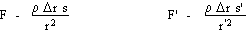...But s
» r2 so that F » F' . If one wants to calculate the value of the gravitational field in a point of a infinite constant matter density field, he must deal with the matter located at infinite distance. Its contribution cannot be neglected.

...Consider a basic problem. We have an infinite distribution of matter, over space, and a single spheric hole. We want to calculate de the field inside. The basic method consist to start from the field due to an infinite, constant density distribution of matter. What does it look like ?

...Simple, says the reader, let's apply the Poisson equation. One computes the flux of the field through a closed surface :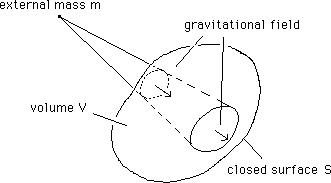Fig. 2 : The flux through a closed surface due to a newtonian field.

then applies the Green theorem :
(3)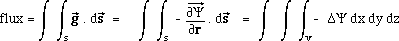Writing :
(4)we get the Poisson equation. We assume that this local law is valid everywhere in space. Then, considering a constant density
r medium, we build the solution :
(4bis)

D Y = 4 p G r = constant

In spherical symmetry :
(5)whose solution is :
(6)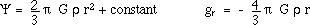...Conclusion : if we take any arbitrary point in space, it is associated to a radial field, which tends to infinite à infinite distance !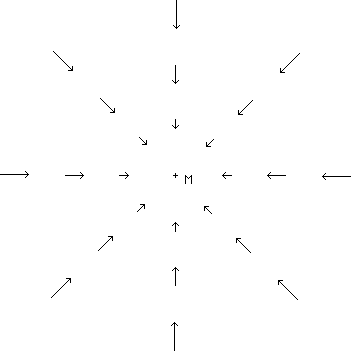Fig.3 : The "classical" gravitational field, in a constant density medium,
around any arbitrarly given point M.

...Isin't strange ? Physically, any given Point P is equally attracted by all the points located in its vicinity. The resultant of the force acting on that point should be zero. If based on that Poisson equation, it is not. Why ?